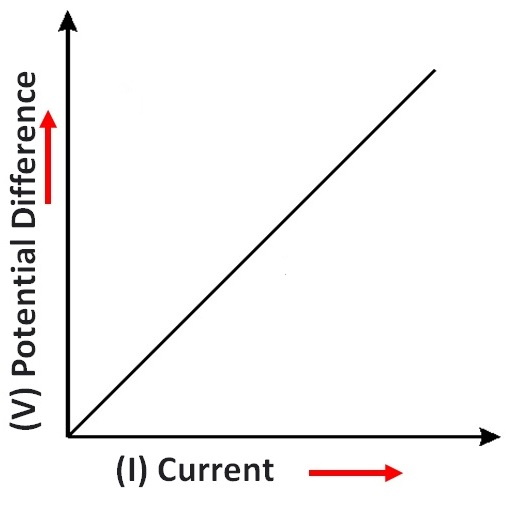Case Based Questions (MCQ)

Class 10
Chapter 12 Class 10 - Electricity

## Observe the graph and answer any four questions from (a) to (e). V-I graph for a conductor is as shown in figure.## (iv) V ∝ 1/I 2

The V-I graph is a straight line graph passing through the origin.

This implies that V is directly proportional to I.

So, the correct answer is (iii ).

## (iv) None of the above

The slope of the V-I graph gives the resistance.

So, the correct answer is (ii).

## (iv) Resistivity

The SI unit of resistance is ohm .

So, the correct answer is (ii).

## (iv) Joule’s Law

Ohm’s law , states that the current passing through a conductor is directly proportional to the potential difference across its ends, provided the physical conditions like temperature, density etc. remain unchanged.

The V-I graph given above also states that current is directly proportional to potential difference.

So, the correct answer is (ii).

## (iv) all of the above

Factors affecting resistance of a conductor are:

• Length of the conductor
• Area of cross section
• Nature of Material
• Temperature

Since all the factors are mentioned in the options.

So, the correct answer is (iv).

Learn in your speed, with individual attention - Teachoo Maths 1-on-1 Class